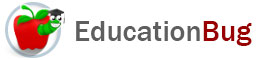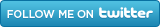PUBLIC SCHOOLS PRIVATE SCHOOLS SCHOOL DISTRICTS COLLEGES PUBLIC LIBRARIES JOBS BLOG RESOURCES
Career Education
Education Choices
Education Costs
Education History and General Information
Education Issues
Education Resources
Family Involvement in Education
Students w Special Needs
Subject Information and Homework Help
Teaching and Learning
Testing
By Types of Schools
By Specific Audiences
GeneralMultiplication

One of the basic math skills everyone needs to know is multiplication. This article defines what multiplication is and how it works, as well as providing information on multiplication tables and how to use them. Keep reading for more on multiplication.

Letâ€™s say you had a number and you had to add it to itself a very large number of times, like this:

16 + 16 + 16 + 16 + 16 + 16 + 16 + 16 + 16 + 16 + 16 + 16 + 16 + 16 + 16  =

Very likely, you would choose to use multiplication instead, and the reason you can do this is that multiplication has the same results as repeated addition.

15 x 16 = is much easier to grasp and much easier to do - either on paper or with a calculator. To understand more about multiplication, keep reading.

The Nomenclature of Multiplication

It is easy enough to connect the name product with the result of multiplication. It may be more difficult, at first, to assign the terms multiplicand - the number to be multiplied - and multiplier, the number that tells the number of repetitions of the multiplicand to the terms one is computing with. Here are some hints to help with this:

1) If you are converting an addition problem to a multiplication problem, it may be easier to understand which element is which. Take the problem above: 16 is the multiplicand and 15 is the multiplier. This is clear because we see that 16 is the number to be multiplied and 15 is the number of multiples.

2) In a horizontal multiplication problem, such as this:

22 x 18 =

- which is read aloud as â€œtwenty-two times eighteen equalsâ€ - the number before the x sign is the multiplier and the number following is the multiplicand.

3) in a vertical multiplication problem, such as this:

111

9 x

the upper number is the multiplicand and the lower number next to the x is the multiplier and you would read this as â€œnine times one hundred eleven.â€

Of course, in some ways it doesnâ€™t matter which number is the multiplicand and which is the multiplier, because 16 x 15 - to return to our first example - has exactly the same product as 15 x 16.,

Multiplication Table

Of course, calculators are useful in many situations for doing quick multiplication, but there are times at which one wants to - or is required to - multiply without them. It was for occasions such as these that the multiplication table, or times table, was invented.

The multiplication table is simply a chart of products of all multipliers and multiplicands from 1 to 10 or sometimes 1 to 12. By memorizing this chart - usually through a variety of tasks, including filling it in a number of times, flash card testing, and the like - student are able to immediately to mind the product of any pair of digits, and can speedily multiply numbers at need - sometimes in their heads.

Here is a multiplication table:

 x 1 2 3 4 5 6 7 8 9 10 1 1 2 3 4 5 6 7 8 9 10 2 2 4 6 8 10 12 14 16 18 20 3 3 6 9 12 15 18 21 24 27 30 4 4 8 12 16 20 24 28 32 36 40 5 5 10 15 20 25 30 35 40 45 50 6 6 12 18 24 30 36 42 48 54 60 7 7 14 21 28 35 42 49 56 63 70 8 8 16 24 32 40 48 56 64 72 80 9 9 18 27 36 45 54 63 72 81 90 10 10 20 30 40 50 60 70 80 90 100

To use the table, you find the multiplier in the first column and the multiplicand in the first row (both shaded gray) and then find the cell at the point of intersection, which will indicate the product. If you prefer to think of the multiplicand in the first column and the multiplier in the first row, that works just as well.

Related Articles
Subtraction